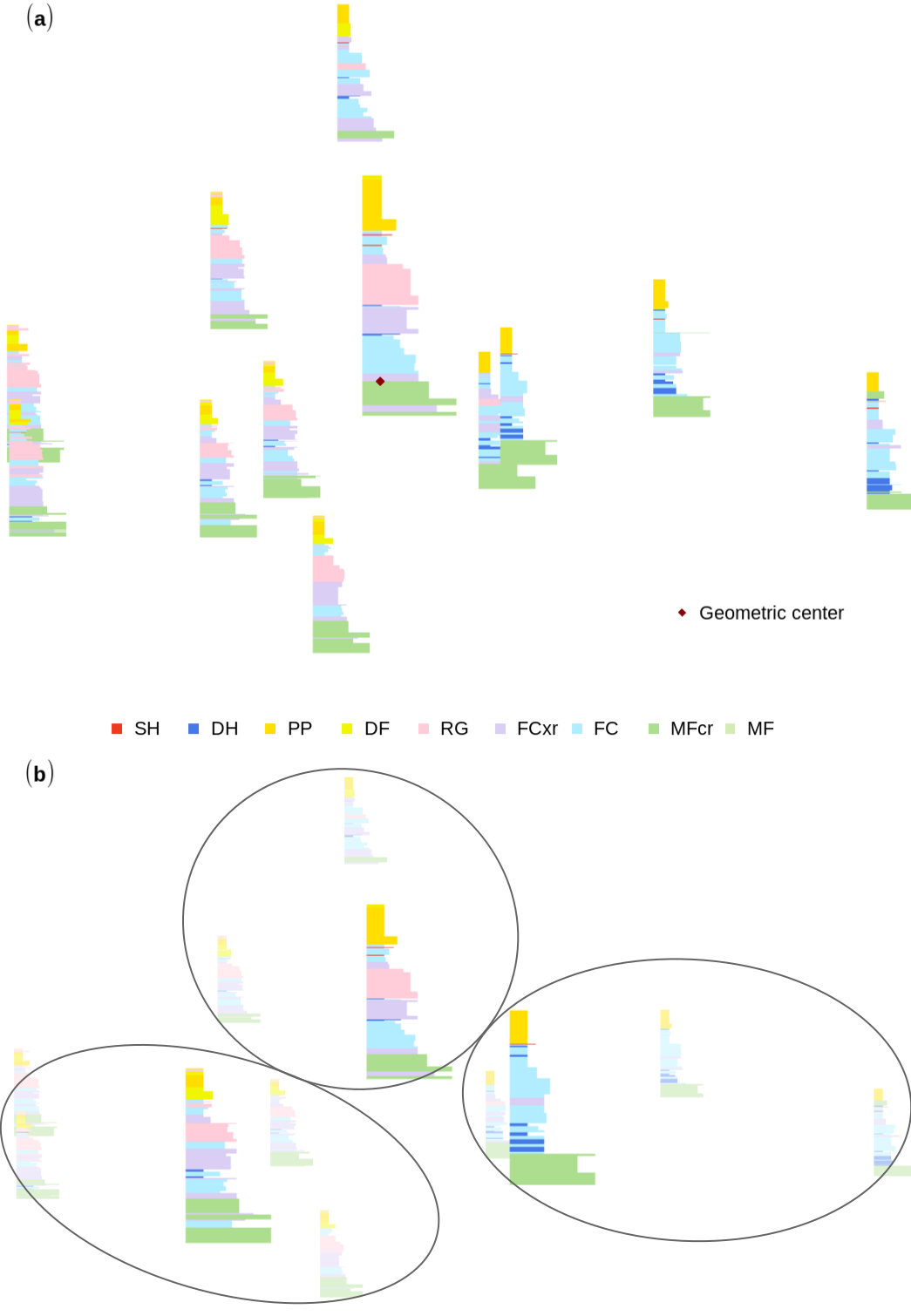# Basic workflow

## Snow profile alignment and similarity assessment for aggregating, clustering, and evaluating of snowpack model output for avalanche forecasting

The package builds on sarp.snowprofile for basic data input/output, profile manipulation and visualization.

This vignette demonstrates the workflow of the analysis methods described in detail in Herla, F., Horton, S., Mair, P., & Haegeli, P. (in review). Snow profile alignment and similarity assessment for aggregating, clustering, and evaluating of snowpack model output for avalanche forecasting.

library(sarp.snowprofile.alignment)

## 1. Aligning snow profiles

Dynamic Time Warping (DTW) can be used to match layers between pairs of snow profiles so that one profile can be warped onto the other profile. That means that the warped profile, which contains the unchanged layer sequences, is optimally aligned to the second profile by adjusting its layer thicknesses.

The core functions for aligning profiles are

• dtwSP: calculate the alignment between two profiles
• plotSPalignment: plot the alignment
• plotCostDensitySP: inspect more details behind the alignment with a cost density plot
## Compute alignment:
dtwAlignment <- dtwSP(SPpairs$A_modeled, SPpairs$A_manual, open.end = FALSE)
## Plot alignment:
plotSPalignment(dtwAlignment = dtwAlignment)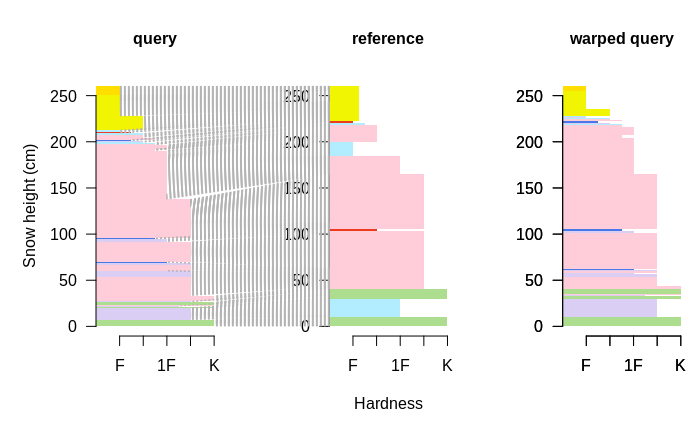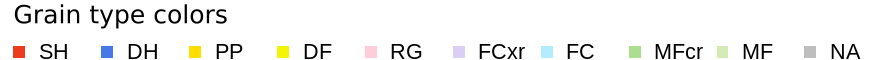Both functions dtwSP and plotSPalignment allow for controlling the settings of the alignment, such as matching of subsequences or constraints to the warping path (i.e., open window size, local slope constraint). See ?dtwSP, or the vignette Technical details for more information.

## Inspect local cost:
plotCostDensitySP(dtwAlignment)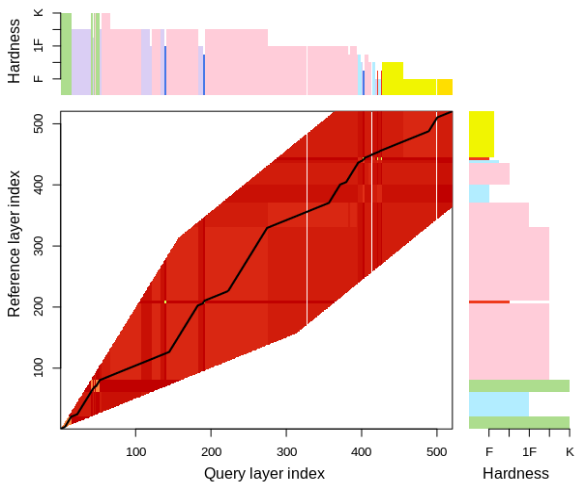The local cost matrix visualizes the distances (i.e., dissimilarities) between individual layer combinations and ultimately shapes the alignment of the profiles through the warping path of least resistance through the matrix.

The local cost matrix can be modified by changing the included layer characteristics (e.g., include/exclude layer date information), by changing the relative weights of the included layer characteristics, or by modifying the preferential layer matching implementation. Read the vignette Technical details for more information.

## 2. Assessing the similarity of profiles

The package provides a function simSP to calculate the similarity between two aligned profiles based on considerations relevant for avalanche hazard assessment. The resulting similarity ranges between [0, 1].

Some alignments require the calculation of that similarity score for choosing the best alignment. In that case, the score can be accessed from the alignment object via $sim. Otherwise, the score can be calculated by dtwAlignment$sim <- simSP(dtwAlignment$reference, dtwAlignment$queryWarped, verbose = TRUE)
#>                wl   cr   pp bulk
#> sim [0, 1]:  0.63 0.88 0.68 0.65
#> simple similarity = 0.711

## 3. Clustering and aggregating profiles

The above methods get even more interesting and useful when applied to sets of snow profiles in order to aggregate or cluster them. Therefor, the package provides medoidSP, which identifies the medoid profile among a set of profiles, and a wrapper function distanceSP (for dtwSP and simSP), which returns the distance between two profiles (i.e., a common input for clustering algorithms).

### 3.1 Clustering

Let’s start with a clustering demonstration of the sample data set SPgroup. You don’t need to, but it is recommended to rescale the set of profiles to identical snow heights before computing a pairwise distance matrix for the profile set.

## rescaling and resampling of the snow profiles:
setRR <- reScaleSampleSPx(SPgroup)$set ## compute the pairwise distance matrix: distmat <- medoidSP(setRR, retDistmat = T)$distmat
#> You are about to compute 12^2 = 144 profile alignments. This will take roughly 43.2 seconds, depending on the profile depths and resampling rate. Starting now..
#> Computed pairwise distance matrix. It actually took
#> Time difference of 26.78318 secs
#> 26.7831778526306

## hierarchichal clustering:
setRR_hcl <- stats::hclust(as.dist(distmat), method = "complete")

The source file to the vignette details how to produce the exact plot based on the cluster object setRR_hcl: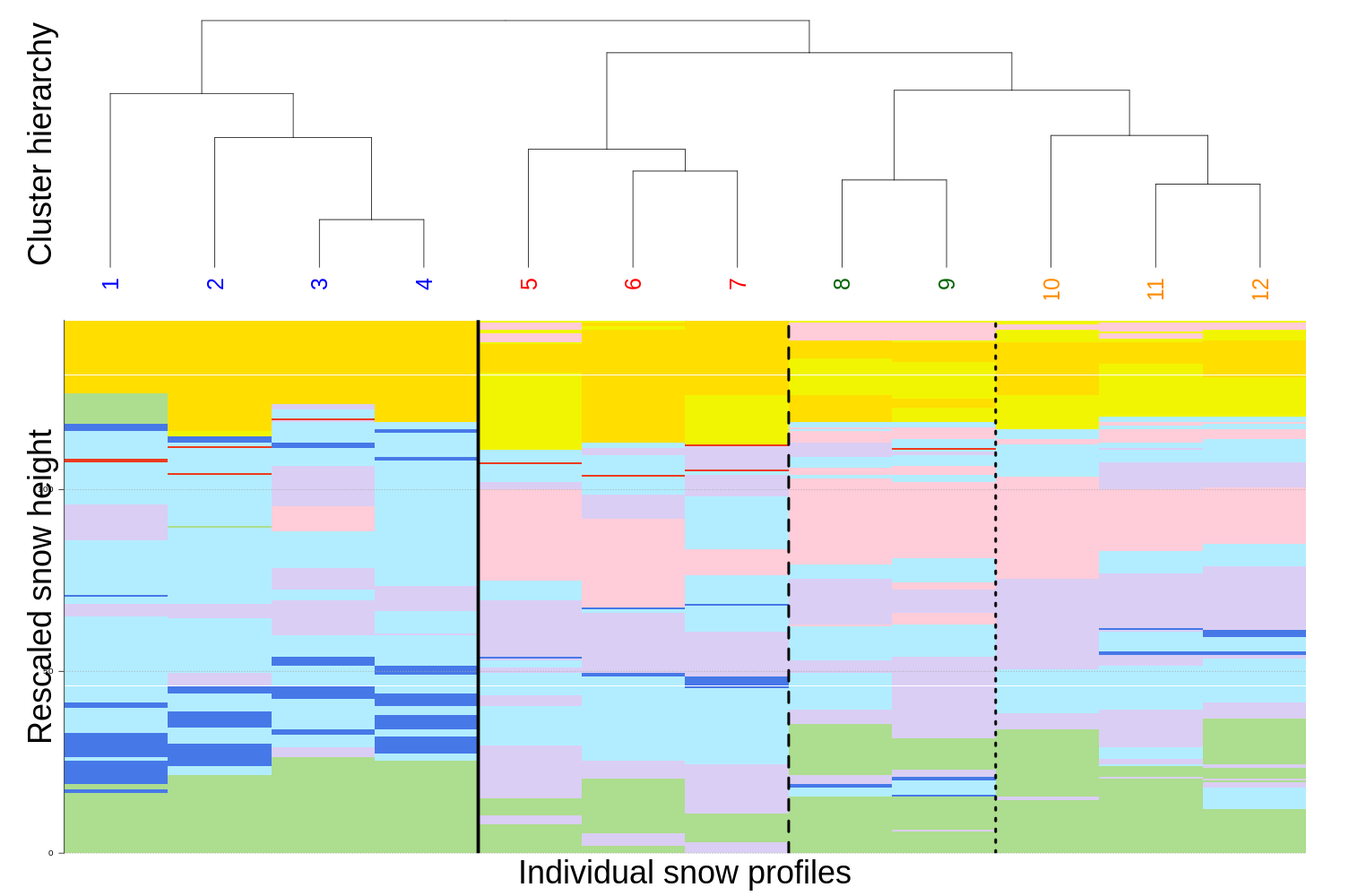Cutting the hierarchical tree to obtain four clusters leads to the colored clusters and the vertical black lines you see in the figure.

### 3.2 Aggregating

The simplest method to aggregating, or summarizing, a set of snow profiles is computing the medoid profile of the set. That medoid profile is the profile with the smallest accumulated distance to all profiles in that set. In other words, it’s the profile closest to the geometric center of the set.

The function medoidSP can be used to conveniently compute the pairwise distance matrix among all profiles (as done above), and to elicit the index of the medoid profile from that distance matrix. The medoid profile of the first (blue) cluster, for example, can be found with

unname(medoidSP(distmat = distmat[1:4, 1:4]))
#>  2

We can visualize the pairwise distances among all profiles in the set with a configuration plot that is based on multidimensional scaling (MDS). That nicely illustrates why a certain profile is supposed to be the medoid profile.

fit <- smacof::mds(as.dist(distmat), type = "ordinal")

Such an MDS fit can be applied to the whole set, or to individual clusters, and can look similar to the following: